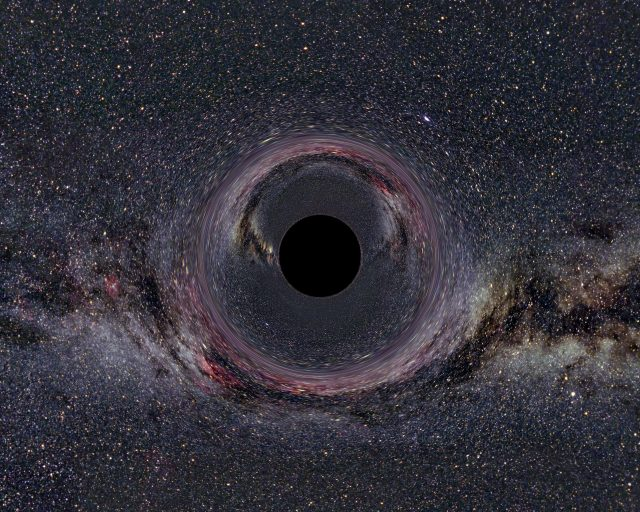# The birth of computational Quantum Gravity?Of all the advances made in theoretical physics in the last twenty years, I still have no doubt that the most impressive one is the so called Maldacena’s conjecture, the guess that the physics involved in some models of quantum gravity living in a very concrete 5-dimensional spacetime has a one-to-one correspondence with the physics predicted by a 4-dimensional Yang-Mills theory (an analogous to the description of strong interactions). This conjecture is so powerful that it has allowed us to describe the physics of strongly coupled quark-gluon plasmas and some other systems that are not tractable in other way, just by using what we have learn about General Relativity in the XX century. And that is because the regimes of the physical theories that are mapped one into the other are not exactly the same.

All quantum theory described by using Feynman’s path integral has three very well defined regimes in which we can study it. First, the theory can be taken to the limit in which all quantum effects are so little that we do not feel them. This limit, formally understood as the limit in which Planck’s constant is taken to zero, is thus called classical limit. By taking it, we recover all the well known predictions of classical mechanics.

However, if we do not take the classical limit and want to study the quantum dynamics of the theory, we can do it in two ways. If the coupling constant of the theory, the physical magnitude that drives the intensity of the interaction (for example, the charge of the electron in electrodynamics or the gravitational constant in gravity), is little, we can expand every physical quantity that we want to measure in powers of this coupling and compute it term by term. This is called perturbative expansion and it is the most well-known tool to compute quantum amplitudes of physical processes.

On the other hand, if the value of the coupling constant is big (and it can be since its value changes with energy), we cannot use the perturbative description and we basically do not know how to compute anything. The only way out is to compute things numerically with a technique called “Lattice field theory” but computations are so heavy that it takes months to calculate the most simple observable even with powerful supercomputers. And this is actually a very big problem, because strong coupled systems contain a lot of very interesting physics, as the dynamics of quark-gluon plasmas or the compositeness of protons. However, some interesting results have been obtained in the last years and things are starting to acquire velocity in this field.

However, in 1998, the argentine physicist Juan M. Maldacena proposed the AdS/CFT conjecture and everything changed. As we have explained here in some occasions, what this conjecture states is that the physics of this quantum theories in the string coupled limit are related to the perturbative expansion of some theory of quantum gravity (originally, the conjecture used a concrete gravitational model that appears in String Theory). It relates the “difficult” region of a theory to the “easy” one of another. It is, thus, an incredibly powerful tool to compute things that cannot be computed in one of them by using the other.

However, until now, all the computations were made in one sense: computing easy things in the gravity side to obtain information about the strong coupled side. The reason for this is that no one can compute more than very few elementary things in the theory of quantum gravity. But this could change at the light of a work recently published in Science magazine1 and written by Masanori Hamada and his collaborators.

What these japanese scientists propose is to use the computation that are already being done by using Lattice Field Theory in a computer to obtain information about the theory of quantum gravity. In other words, if we trust that the conjecture is true (and no physicist doubt about this) lattice computations can be used to explore the physics of quantum gravity, and this is a big step into the understanding of this still unknown theory. If it is really possible, this is the dawn of computational quantum gravity.

So, what Hamada et al. do in their paper is to show that one of the few quantities that one can easily compute in quantum gravity (related to the behavior of the horizon of a black hole) can be as well obtained by taking the adequate computer calculation and using the dictionary of the conjecture. Therefore, we have reasons to trust that this also happens with any other quantity, even with the ones we do not know how to compute in the gravity side.

Personally, I would not say that this work is revolutionary in the sense that it discovers a new physics, but it a proof that one can put together all the little pieces that we have about quantum gravity and the puzzle works. Of course, this is restricted to a particular model of quantum gravity, which maybe it is not the one describing the world we are living in, and moreover it is also restricted to a very particular spacetime, so it is not useful to do computations about our real world. However, having information about how a quantum gravity is can be very useful to understand how the quantum gravity is (it could be specially interesting to understand some physics related to the exotic dark energy). And in physics, everything is about understanding.

## References

1. Hanada M., Hyakutake Y., Ishiki G. & Nishimura J. Holographic Description of a Quantum Black Hole on a Computer, Science, DOI: 10.1126/science.1250122